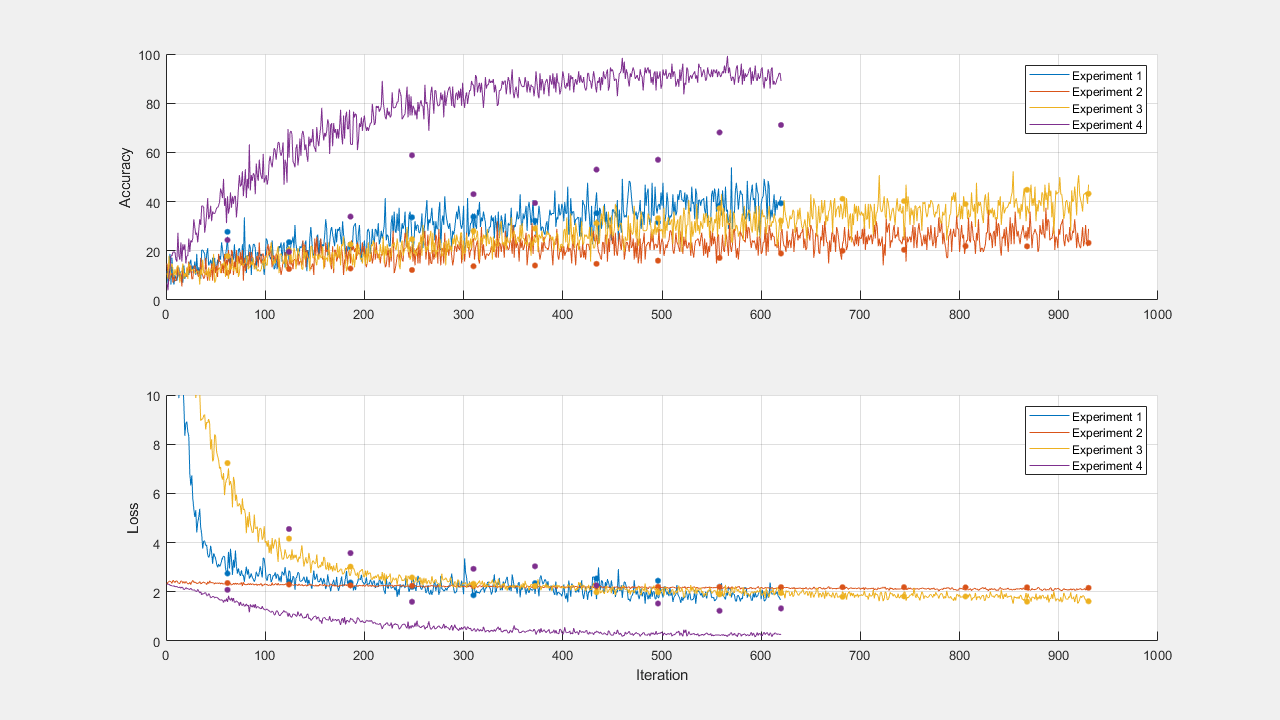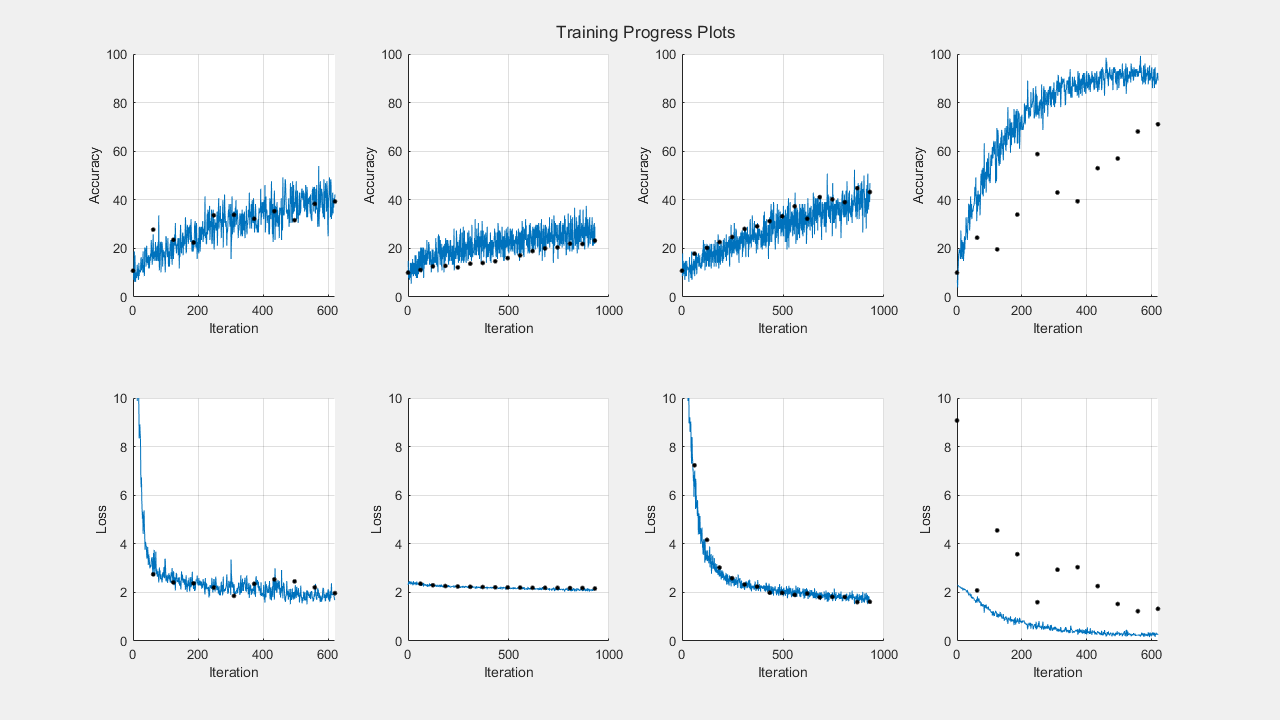# 并行训练深度学习网络

### 准备数据集

```datasetLocation = fullfile(matlabroot,'toolbox','nnet', ... 'nndemos','nndatasets','DigitDataset');```

• 将数据集上传到 Amazon S3 存储桶中。有关示例，请参阅将深度学习数据上传到云

• 创建一个云集群。在 MATLAB 中，您可以直接通过 MATLAB 桌面在云中创建集群。有关详细信息，请参阅Create Cloud Cluster (Parallel Computing Toolbox)

• 选择您的云集群作为默认集群，在主页选项卡的环境部分中，选择 Parallel > Select a Default Cluster

### 加载数据集

```imds = imageDatastore(datasetLocation, ... 'IncludeSubfolders',true, ... 'LabelSource','foldernames'); [imdsTrain,imdsValidation,imdsTest] = splitEachLabel(imds,0.8,0.1);```

```imageSize = [28 28 1]; pixelRange = [-4 4]; imageAugmenter = imageDataAugmenter( ... 'RandXReflection',true, ... 'RandXTranslation',pixelRange, ... 'RandYTranslation',pixelRange); augmentedImdsTrain = augmentedImageDatastore(imageSize,imdsTrain, ... 'DataAugmentation',imageAugmenter);```

### 并行训练网络

```numGPUs = gpuDeviceCount("available"); parpool(numGPUs);```
```Starting parallel pool (parpool) using the 'local' profile ... Connected to the parallel pool (number of workers: 2). ```

`dataqueue = parallel.pool.DataQueue;`

`[layersCell,options] = networkLayersAndOptions(augmentedImdsTrain,imdsValidation,dataqueue);`

`handles = preparePlots(numel(layersCell));``afterEach(dataqueue,@(data) updatePlots(handles,data));`

`trainingFuture(1:numel(layersCell)) = parallel.FevalFuture;`

```for i=1:numel(layersCell) trainingFuture(i) = parfeval(@trainNetwork,2,augmentedImdsTrain,layersCell{i},options(i)); end```

`parfeval` 不会阻止 MATLAB，因此您可以在计算的同时继续工作。

`[network,trainingInfo] = fetchOutputs(trainingFuture);````filename = sprintf('experiment-%s',datetime('now','Format','yyyyMMdd''T''HHmmss')); save(filename,'network','trainingInfo');```

### 绘制结果

```figure('Units','normalized','Position',[0.1 0.1 0.6 0.6]); title('Training Progress Plots'); for i=1:numel(layersCell) subplot(2,numel(layersCell),i); hold on; grid on; ylim([0 100]); iterationsPerEpoch = floor(augmentedImdsTrain.NumObservations/options(i).MiniBatchSize); epoch = (1:numel(trainingInfo(i).TrainingAccuracy))/iterationsPerEpoch; plot(epoch,trainingInfo(i).TrainingAccuracy); plot(epoch,trainingInfo(i).ValidationAccuracy,'.k','MarkerSize',10); end subplot(2,numel(layersCell),1), ylabel('Accuracy');```

```for i=1:numel(layersCell) subplot(2,numel(layersCell),numel(layersCell) + i); hold on; grid on; ylim([0 max([trainingInfo.TrainingLoss])]); iterationsPerEpoch = floor(augmentedImdsTrain.NumObservations/options(i).MiniBatchSize); epoch = (1:numel(trainingInfo(i).TrainingAccuracy))/iterationsPerEpoch; plot(epoch,trainingInfo(i).TrainingLoss); plot(epoch,trainingInfo(i).ValidationLoss,'.k','MarkerSize',10); xlabel('Epoch'); end subplot(2,numel(layersCell),numel(layersCell)+1), ylabel('Loss');```